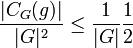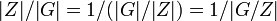Commuting fraction more than five-eighths implies abelian

This article is about a result whose hypothesis or conclusion has to do with the fraction of group elements or tuples of group elements satisfying a particular condition.The fraction involved in this case is 5/8
View other such statements

History

It is likely that this statement, being quite simple, was known to many people since the beginnings of group theory and/or rediscovered a number of times. The first written proof appears in a paper by Erdos and Turan.

Statement

For a finite group in terms of commuting fractions

Suppose$G$ is a finite group such that the commuting fraction of$G$ is more than$5/8$. Then,$G$ is an abelian group, and in particular, a finite abelian group.

In particular, if we define:$CP(G) := \{ (x,y) \in G^2 \mid xy = yx \}$

Then:$\! \frac{|CP(G)|}{|G|^2} > \frac{5}{8} \implies G$ abelian

Note that since the commuting fraction of a finite abelian group is$1$, this means that the commuting fraction of a finite group cannot take any value strictly between$5/8$ and$1$.

For a finite group in terms of conjugacy classes

Suppose$G$ is a finite group and$n(G)$ is the number of conjugacy classes in$G$. Then:$\frac{n(G)}{|G|} > \frac{5}{8} \implies G$ abelian.

For a FZ-group

Suppose$G$ is a FZ-group whose commuting fraction is more than$5/8$. Then,$G$ is an abelian group.

Related facts

Applications

The fact has applications to the abelianness testing problem, and is the basis for the randomized black-box group algorithm for abelianness testing.

Proof

Proof for a finite non-abelian group

Given: A finite non-abelian group$G$.

To prove: The commuting fraction of$G$ is at most$5/8$.

Proof: Let$Z$ be the center of$G$.

Step no. Assertion Given data used Facts used Previous steps used Explanation
1$G/Z$ is not cyclic$G$ is non-abelian Fact (1) -- By fact (1), if$G/Z$ were cyclic,$G$ would be abelian, a contradiction.
2$|G/Z|$ is at least$4$ -- Step (1) Any group of order 1, 2, or 3 is cyclic, forcing$G/Z$ to have size at least$4$.
3 For all elements$g$ in$G$ and not in$Z$, the quotient$|C_G(g)|/|G|$ is at most$1/2$ Fact (2) -- If$g$ is not in$G$, then$C_G(g)$ is a proper subgroup of$G$. By fact (2), we get that$|C_G(g)|/|G| \le 1/2$.
4 The commuting fraction of$G$ is$\frac{|Z|}{|G|} + \sum_{g \in G\setminus Z} \frac{|C_G(g)|}{|G|^2}$ -- -- By definition. Explicitly, the commuting fraction is$\sum_{g \in G} \frac{|C_G(g)|}{|G|^2}$. Those$g$ that are in$Z$ can be separated out and we get the summation in the form presented.
5 The commuting fraction of$G$ is at most$\frac{|Z|}{|G|} +\frac{(|G| - |Z|)}{|G|} \frac{1}{2}$ -- Steps (3) and (4) By Step (3),$\frac{|C_G(g)|}{|G|^2} \le \frac{1}{|G|} \frac{1}{2}$ for each$g \in G \setminus Z$. The sum is thus bounded by the size of$G \setminus Z$ times this individual bound.
6 The commuting fraction of$G$ is at most$\frac{1}{2} + \frac{|Z|}{2|G|}$ -- Step (5) Algebraic simplification
7 The commuting fraction of$G$ is at most$5/8$ Fact (2) Steps (2), (6) By Fact (2),$|Z|/|G| = 1/(|G|/|Z|) = 1/|G/Z|$ which by Step (2) is at most 1/4. Plugging this into Step (6), we get that the commuting fraction of$G$ is at most$\frac{1}{2} + \frac{1}{2} \frac{1}{4} = \frac{5}{8}$.